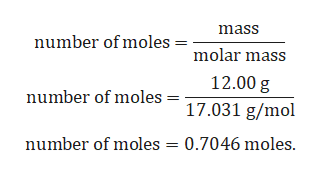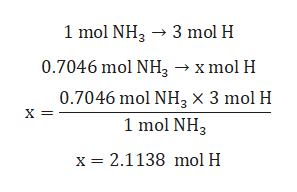# How do you calculate the mass of Hydrogen in 12.00 g of NH3?

Question

How do you calculate the mass of Hydrogen in 12.00 g of NH3?

check_circle

Step 1

Given:

Mass of NH3 = 12.00 g.

Molar mass of NH3 = 17.031 g/mol.

Step 2

Calculation for number of moles of NH3:help_outlineImage Transcriptionclosemass number of moles molar mass 12.00 g number of moles = 17.031 g/mol number of moles = 0.7046 moles. fullscreen
Step 3

Calculation for number of moles of H:

1 mole of ...help_outlineImage Transcriptionclose1 mol NH33 mol H 0.7046 mol NH3 xmol H 0.7046 mol NH3 x 3 mol H 1 mol NH3 X = x 2.1138 mol H fullscreen

### Want to see the full answer?

See Solution

#### Want to see this answer and more?

Solutions are written by subject experts who are available 24/7. Questions are typically answered within 1 hour.*

See Solution
*Response times may vary by subject and question.
Tagged in

### General Chemistry